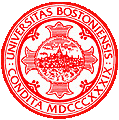# Programming Assignment 2

CS 585 HW 2
Kaiyuan Fan
09/26/2018

## Problem Definition

The problem of this assignment is to design and implement algorithms that can analyze and recognize random shapes. There are few requirements.

1. For each shape image, determine its background color and label each and every shape blobs according to their color.
2. For each shape blob, implement a border following algorithm to find its outermost contour (i.e. the border pixels) and compare it with OpenCV's "findContours" function.
3. For each shape blob, classify its border pixels (in other words, segment its outermost contour) into three types: borders against the background, borders against another shape blob and borders that are a border of the whole image.
4. For each shape blob, come up with an algorithm that can recognize its shape type (square, circle, or triangle).

## Method and Implementation

1. In order to determine its background color, I store the colors of all the pixels into a vector of subvectors, the biggest size of subvector will be the color of background. And the other colors will be the color of blobs. I am assuming the colors are consistent. (I am using the optimized data)
2. In the first requirement, I have store all blobs in a vector, in order to find the contours I search by each pixel's 8 neighbors and return different value according it's neighbor's color.
3. With the different return value, I can segment the contour if it at a border or if it against other shapes.
4. I first determine if an object is a square according to if the shape contour contains a vertical straight line (by counting the frequency of Ys in contour pixels(X, Y)), if Y exceed 20% of the object contour. size, I determine the object is a square. Then if the shape has a horizontal line, if it does, I determine the shape as a triangle. Otherwise, the shape is a circle.

Functions: void labelShapes(Mat& labelled, vector <vector<Point2i>> &blobs); void findShapes(Mat &image, vector <vector<Point2i>> &blobs); void findContours(Mat &image, vector <vector<Point2i>> &blobs, vector <vector<Point2i>> &contours); int checkNeighbor(Mat &image, int &c, int &r); void labelContours(Mat &image, Mat &labelled, vector <vector<Point2i>> &contours); void detectShapes(vector <vector<Point2i>> &contours); int checkShape(int &size, int &max_x, int &max_y);

## Experiments

During this assignment, I have tried around 40 experiments. I am using the optimized data. Overall since the shapes are not complex, I got almost over 90% of correct result for all shapes. Below is an example of my program running image with 4 shapes.

Because my program fully operated on the pixels colors, so it doesn't work in orignal data. I labelled first object as blue, second as green, third as red, and fourth as yellow. Also, the contour at border as white, contour that against each other as orange.

## Results### Results

Trial Source Image Labelled Image Contours Image Shape Type
example 1example 2## Discussion

Discuss your method and results:

As we can see in example 1, my program can correctly recognize the shapes. But in example 2, shapes are overlapping, the horizontal line of the triangle is covered by the square, it causes my program to consider the triangle as a circle. The fourth shape is also a triangle, but the vertical line of square confused my program. In the future, I can solve these shortcomings to improve the efficiency of my program.

## Conclusions

In conclusion, My program can correctly handle most of the images, but in some special cases, my program cannot distinguish the different shapes of objects. During this assignment, I have learned how to create own methods of function other than using build-in functions in OpenCv.

## Credits and Bibliography

Got the idea of recognizing shapes at yifu's office hours.